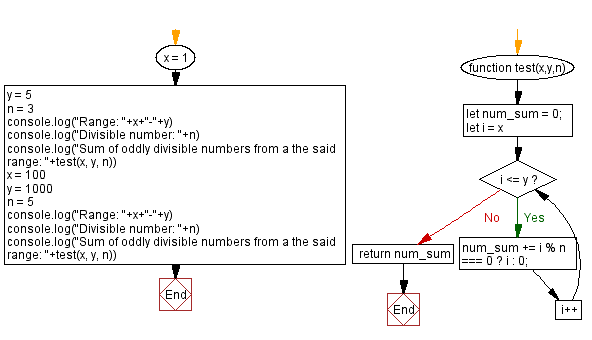# JavaScript: Sum of oddly divisible numbers from a range

## JavaScript Math: Exercise-89 with Solution

Write a JavaScript program that takes three arguments x, y, n and calculates the sum of the numbers oddly divided by n from the range x, y inclusive.

Test Data:
(1,5,3) -> 3
(100, 1000, 5) -> 99550

Sample Solution:

HTML Code:

``````<!DOCTYPE html>
<html>
<meta charset="utf-8">
<title>JavaScript program to Sum of oddly divisible numbers from a range</title>
<body>

</body>
</html>
```
```

JavaScript Code:

``````function test(x,y,n) {
let num_sum = 0;
for (let i = x; i <= y; i++) {
num_sum += i % n === 0 ? i : 0;
}
return num_sum
}
x = 1
y = 5
n = 3
console.log("Range: "+x+"-"+y)
console.log("Divisible number: "+n)
console.log("Sum of oddly divisible numbers from a the said range: "+test(x, y, n))
x = 100
y = 1000
n = 5
console.log("Range: "+x+"-"+y)
console.log("Divisible number: "+n)
console.log("Sum of oddly divisible numbers from a the said range: "+test(x, y, n))
```
```

Sample Output:

```Range: 1-5
Divisible number: 3
Sum of oddly divisible numbers from a the said range: 3
Range: 100-1000
Divisible number: 5
Sum of oddly divisible numbers from a the said range: 99550
```

Flowchart:Live Demo:

See the Pen javascript-math-exercise-89 by w3resource (@w3resource) on CodePen.

Improve this sample solution and post your code through Disqus

What is the difficulty level of this exercise?

Test your Programming skills with w3resource's quiz.

﻿